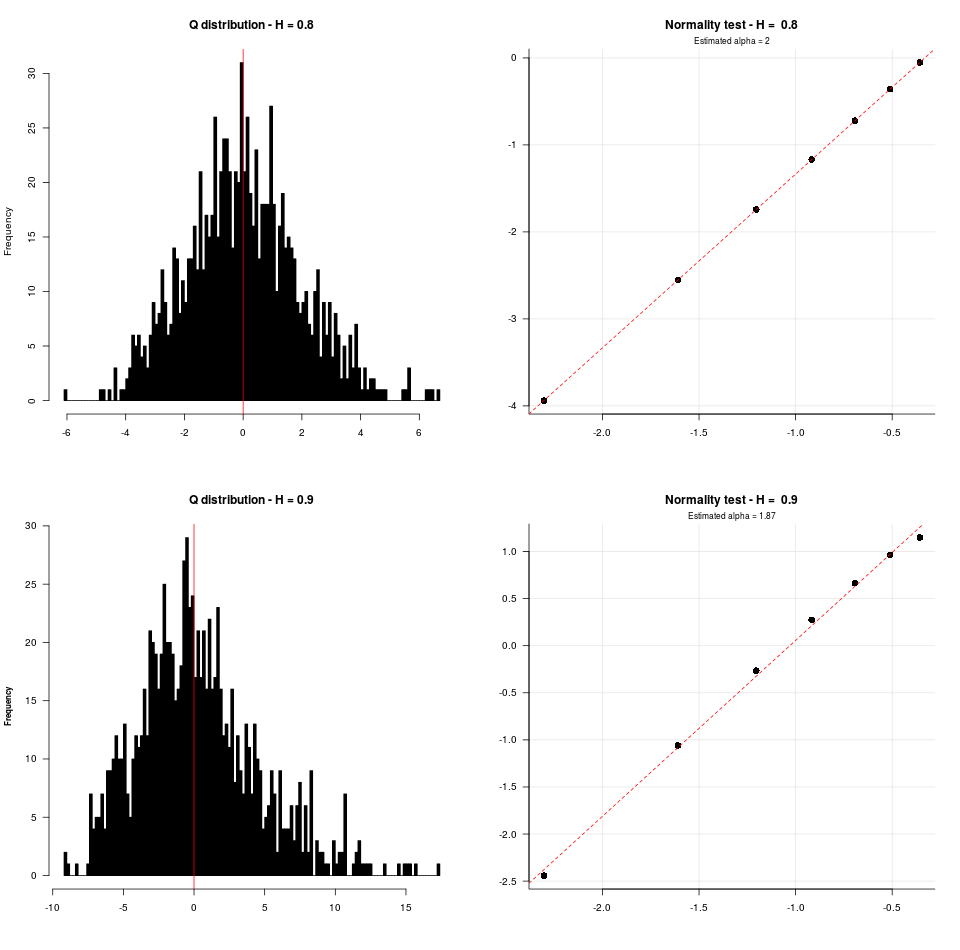# Appendix D - Properties of the estimators

By Mariachiara Fortuna | April 24, 2020

Affiliations:

John K. Dagsvik, Statistics Norway, Research Department;

Mariachiara Fortuna, freelance statistician, Turin;

Sigmund Hov Moen, Westerdals Oslo School of Arts, Communication and Technology.

Corresponding author:

John K. Dagsvik, E-mail:

Mariachiara Fortuna, E-mail: (reference for code and analysis)

### Table D1, D2, D3. Properties of different estimators of the FGN model. Bootstrap simulations

The following tables show the results of the bootstrap simultations for different estimators of $\mu$, $\sigma$, $H$ and $\alpha$, given the FGN model with H equal to 0.7, 0.8, 0.9, 0.95.

Each bootstrap estimate is based on 1,000 simulated FGN series of length 2,000.

### Table D1. Results of the bootstrap simulations for estimators of $\mu$ and $\sigma$

• $\mu_c$ is the characteristic function estimator for the mean, and $SE(\mu_c)$ is its bootstrap simulation standard error

• $\mu_{ML}$ is the maximum likelihood estimator for the mean, and $SE(\mu_{ML})$ is its bootstrap standard error

• $\sigma_c$ is the characteristic function estimator for the standard deviation, and $SE(\sigma_{ML})$ is its bootstrap standard error

• $\sigma_{ML}$ is the maximum likelihood estimator for the standard deviation, and $SE(\sigma_{ML})$ is its bootstrap standard error

H $\mu_c$ $SE(\mu_c)$ $\mu_{ML}$ $SE(\mu_{ML})$
0.70 0.002 0.002 0.002 0.002
0.80 -0.001 -0.001 -0.001 -0.001
0.90 -0.012 -0.012 -0.011 -0.011
0.95 -0.024 -0.024 -0.021 -0.021
H $\sigma_c$ $SE(\sigma_c)$ $\sigma_{ML}$ $SE(\sigma_{ML})$
0.70 0.994 0.039 0.990 0.039
0.80 0.956 0.053 0.950 0.056
0.90 0.793 0.080 0.789 0.086
0.95 0.535 0.073 0.534 0.077

The results in Table D1 show that the standard errors of the respective estimators for the mean increase substantially when H increases. Also the estimators for $\sigma$ become severely downward biased.

### Table D2. Bootstrap simulations results for the H parameter

• $H_c$ is the characteristic function estimator for the H parameter, and $SE(H_c)$ is its bootstrap standard error

• $H_w$ is the Whittle estimator for the the H parameter, and $SE(\mu_{ML})$ is its bootstrap standard error

H $H_c$ $SE(H_c)$ $H_{ML}$ $SE(H_{ML})$
0.70 0.693 0.019 0.700 0.015
0.80 0.781 0.021 0.799 0.015
0.90 0.860 0.023 0.899 0.015
0.95 0.891 0.021 0.947 0.014

Table D2 shows that the characteristic function estimator becomes downward biased as H increases.

### Table D3. Bootstrap simulations results for the $\alpha$ parameter of the stable distribution

$\alpha_c$ is the characteristic function estimator for the $\alpha$ parameter, and $SE(\alpha_c)$ is its bootstrap standard error

H $\alpha_c$ $SE(\alpha_c)$
0.70 2 0.003
0.80 2 0.002
0.90 2 0.001
0.95 2 0.001

The results in Table D3 show that the characteristic function estimator in this case performs very well at any level of H.

### Figure D1 and D2. Graphical test of asymptotic normality of the characteristic function estimators

Bootstrap estimates based on 1,000 simulated FGN series of length 2,000.

## Figure D3. Graphical test of the distribution of the Chi-Square statistics Q when estimated H values are inserted

Bootstrap estimates based on 1,000 simulated FGN series of length 2,000.

H estimated by the Whittle method.Figure D3 shows that Q is approximately normally distributed when H = 0.8 whereas the distribution becomes skew to the right when H = 0.9. (similar to a stable distribution that is totally skew to the right)